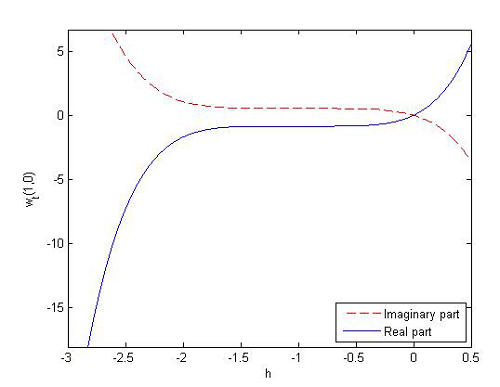• P-ISSN 0974-6846 E-ISSN 0974-5645# Indian Journal of Science and Technology

## Article• VIEWS 766
• PDF 205

Indian Journal of Science and Technology

Year: 2020, Volume: 13, Issue: 24, Pages: 2387-2403

Original Article

## Solving the cubic complex Ginzburg-Laundau equation by Homotopy analysis method

Received Date:22 May 2014, Accepted Date:26 July 2015, Published Date:09 July 2020

## Abstract

Objectives: This paper obtains the series solution of the cubic complex Ginzburg-Laundau equation, by means of homotopy analysis method(HAM). Methods: In addition to the homotopy analysis method, homotopy perturbation and Adomian decomposition methods are applied to determine approximation solution of the cubic complex Ginzburg-Laundau equation and advantage of using HAM. Also a theorem is proved to guarantee the convergence of the HAM to solve this equation. Findings: Three examples are solved to illustrate the efficiency of the proposed method, this method is compared with other analytical approximate methods such as homotopy perturbation method (HPM)and Adomiam decomposition method(ADM) and it can be seen that these methods have the same results for this equation. Application: Homotopy analysis method as a reliable and valid scheme can be used to work out the cubic complex Ginzburg-Laundau equation which is nonlinear partial differential equation.

Keywords: Homotopy analysis method; Ginzburg-Laundau

## References

1. Eguiluz V, Hernandez-Garcia E, Piro O. Boundary effects in the complex Ginzburg- Landau equation. International Journal of Bifurcation and Chaos in Applied Sciences and Engineering. 1999;9(11):2209–2214.
2. Charbonneau G. De l’hysérie masculine : Comprendre l’hysérie par son expression masculine. In: PSN. (Vol. 4, pp. 6-10) Vancouver, B.C. Canada. Springer Science and Business Media LLC. 2006.
3. Ginibre J, Velo G. The Cauchy Problem in Local Spaces for the Complex Ginzburg--Landau Equation¶II. Contraction Methods. Communications in Mathematical Physics. 1997;187(1):45–79. Available from: https://dx.doi.org/10.1007/s002200050129
4. Gao H, Wang X. On the global existence and small dispersion limit for a class of complex Ginzburg-Landau equations. Mathematical Methods in the Applied Sciences. 2009;32:1396–1414.
5. Saarloos WV. The Complex Ginzburg-Landau equation for beginners. (Vol. 9506) Leiden The Netherlands. 1994.
6. Yang Y, Gao H. Continuous dependence on modelling for a complex Ginzburg Landau equation with complex coefficients. Mathematical Methods in the Applied Sciences. 2004;27:1567–1578.
7. Marcq P, Chaté H, Conte R. Exact solutions of the one-dimensional quintic complex Ginzburg-Landau equation. Physica D: Nonlinear Phenomena. 1994;73(4):305–317. Available from: https://dx.doi.org/10.1016/0167-2789(94)90102-3
8. Aranson IS, Kramer L. 2001.
9. Nasibov S. On a class of non-linear evolution Ginzburg-Landau-Schrodinger type equation. Applied and computational mathematics. 2004;3(2):142–151.
10. Sherratt JA, Smith MJ, Rademacher JDM. Patterns of Sources and Sinks in the Complex Ginzburg–Landau Equation with Zero Linear Dispersion. SIAM Journal on Applied Dynamical Systems. 2010;9(3):883–918. Available from: https://dx.doi.org/10.1137/090780961
11. Wazwaz A. 2009.
12. Liao SJ. 1992.
13. Alizadeh-Pahlavan A, Aliakbar V, Vakili-Farahani F, Sadeghy K. MHD flows of UCM fluids above porous stretching sheets using two-auxiliary-parameter homotopy analysis method. Communications in Nonlinear Science and Numerical Simulation. 2009;14(2):473–488. Available from: https://dx.doi.org/10.1016/j.cnsns.2007.09.011
14. Alomari AK, Noorani MSM, Nazar R. Explicit series solutions of some linear and nonlinear Schrodinger equations via the homotopy analysis method. Communications in Nonlinear Science and Numerical Simulation. 2009;14:1196–1207. Available from: https://dx.doi.org/10.1016/j.cnsns.2008.01.008
15. Alomari AK, Noorani MSM, Nazar R. Comparison between the homotopy analysis method and homotopy perturbation method to solve coupled Schrodinger-KdV equation. Journal of Applied Mathematics and Computing. 2009;31(1-2):1–12. Available from: https://dx.doi.org/10.1007/s12190-008-0187-4
16. Fariborzi Araghi MA, Naghshband S. On convergence of homotopy analysis method to solve the Schrodinger equation with a power law nonlinearity. International Journal of Industrial Mathematics. 2013;5(4):367–374.
17. Naghshband S, Araghi MAF. Approximation solution of the complex modified Korteweg-de Vries equation via the homotopy analysis method. Communications on Advanced Computational Science with Applications. 2017;2017(1):12–23. Available from: https://dx.doi.org/10.5899/2017/cacsa-00066
18. Naghshband S, Araghi F, MA. Solving generalized quintic complex Ginzburg-Landau equation by homotopy analysis method. Ain Shams Engineering Journal. 2016.
19. Fariborzi Araghi MA, Fallahzadeh A. On the convergevce of the homotopy analysis method for solving the Schrodinger equation. Journal of Basic and Applied Scientific Research. 2012;(2) 6076–6083.
20. Biazar J, Ansari R, Hosseini K, Gholamian P. 2008.
21. Wazwaz A. A new algorithm for calculating adomian polynomials for nonlinear operators. Applied mathematics and computation. 2000;111:53–69.
22. Liao SJ. Beyond perturbation:Introduction to the homotopy analysis method. Boca Raton. Chapman and Hall/CRC Press. 2003.
23. Biazar J, Partovi M, Ayati Z. Approximating Solutions for Ginzburg- Landau Equation by HPM and ADM. Applications and Applied Mathematics. 2010;p. 1672–1681.Article Metrics